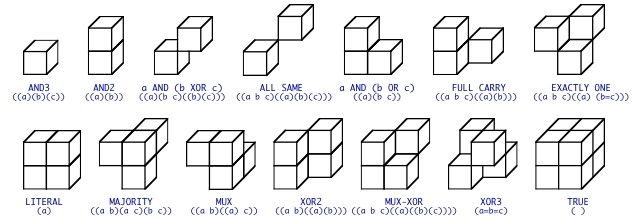# Boolean Cubes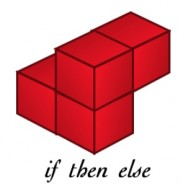A Boolean function is a relationship between variables that can take on one of two values, {0,1}. The Boolean operators are {AND, OR, NOT}. George Boole’s great contribution, in his 1854 book The Laws of Thought, was to show that the logic embedded in language can be expressed as an algebraic system. Boolean logic can also be expressed as spatial arrangements of squares (in the case of the 16 two-variable functions) or cubes (in the case of the 256 three-variable functions). Iconic containment forms expose the structure and meaning of Boolean cubes. The Image, for example, shows the spatial structure of the three-variable IF-THEN-ELSE function, if A then B else C. It’s corresponding containment form is (((A) B)(A C)). The number of Boolean functions can be reduced (abstracted) by identifying the same spatial forms that are invariant under change of viewpoint. Viewing perspective transformation of objects in space are translations, rotations and reflections. Spatial symmetries reduce the 16 two-variable functions to four distinguishable forms, and the 256 three-variable functions to fourteen forms. The simplification factor for three variable functions is 18 to 1; 295 to 1 for four-variable functions; and 6971 to 1 for five-variable functions. Spatial symmetry reduces the IF-THEN-ELSE in the image from twelve varieties to one.

A greater gain is that the spatial configuration of cubes shows how particular functions can be simplified further. Cubes with faces and edges that touch can be simplified. The IF-THEN-ELSE in the image has three shared faces; its generic expanded form with 24 variable occurrences reduces to 4 variable occurrences. Spatial cubes are visual, iconic tools that simplify complicated symbolic logical expressions. They are also visual analogs of Boolean matrices.

The article Symmetry in Boolean Functions with Examples for Two and Three Variables includes a discussion of these structures, with emphasis on the 256 varieties of three-variable Boolean functions.

### 2D Boolean Cubes

Here are the sixteen Boolean cubes for two variables.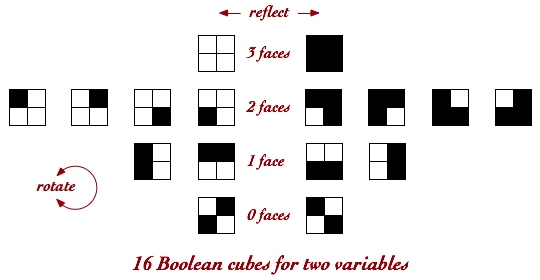There are four varieties and their reflections. Reflections of the zero- and one-face varieties are also rotations. For comparison here are the sixteen Venn diagrams for two variables.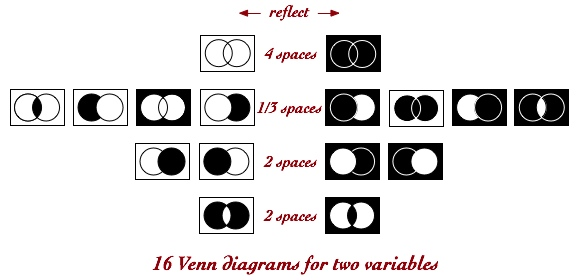The position of each Boolean cube corresponds to the same position in the Venn diagrams. However the rotational symmetries are lost. For comparison, here are the sixteen parens forms for two variables, in corresponding locations.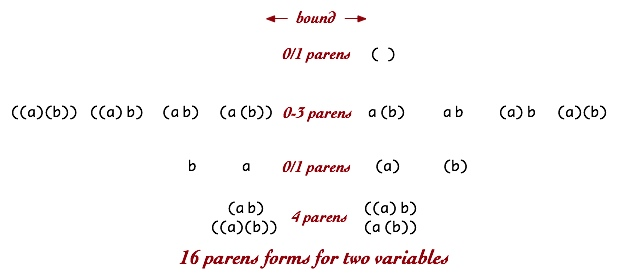Again the structural emphases change. bounding does not align with reflections, parens nesting does not align with faces or spaces. Each of these different organizational approaches highlights different aspects of the same abstract Boolean two-variable structure. None correspond to the group theoretic organization of two-variable Boolean functions as a complemented, distributed lattice in 4-space.

### 3D Boolean Cubes

There are the 256 Boolean cubes for three variables. These cubes can be represented as three-dimensional objects. When symmetries and reflections are ignored, the 256 varieties reduce to the fourteen shown below. Each of these configurations is labeled by the parens structure that identifies them.